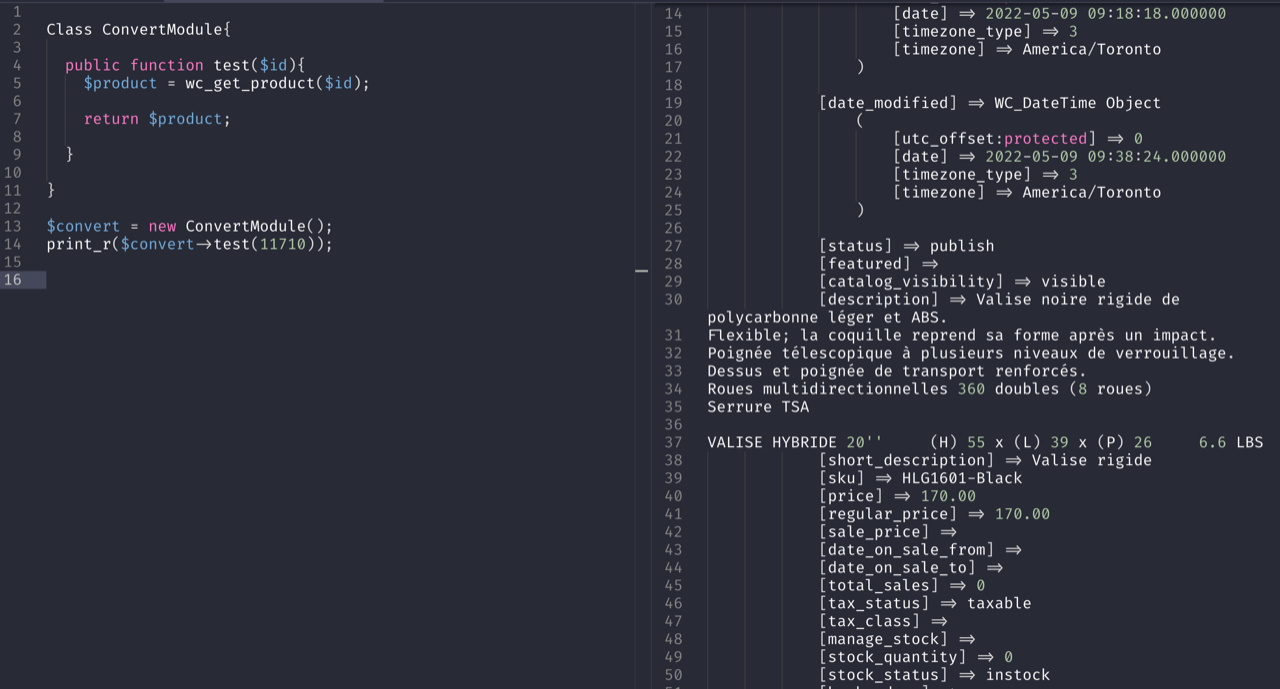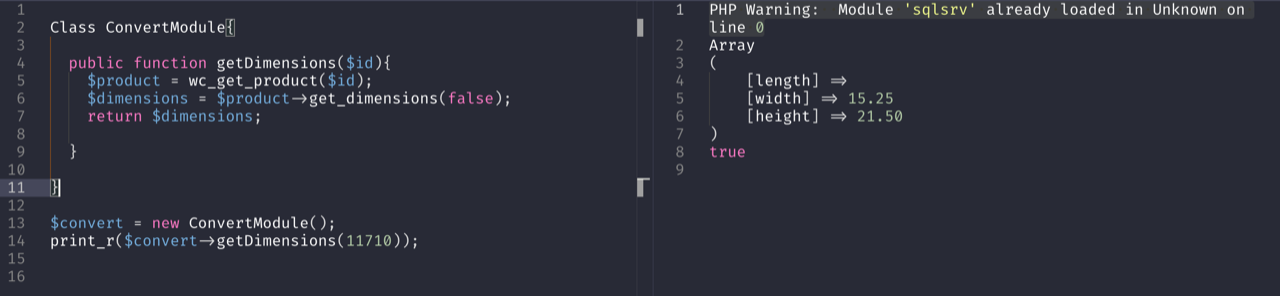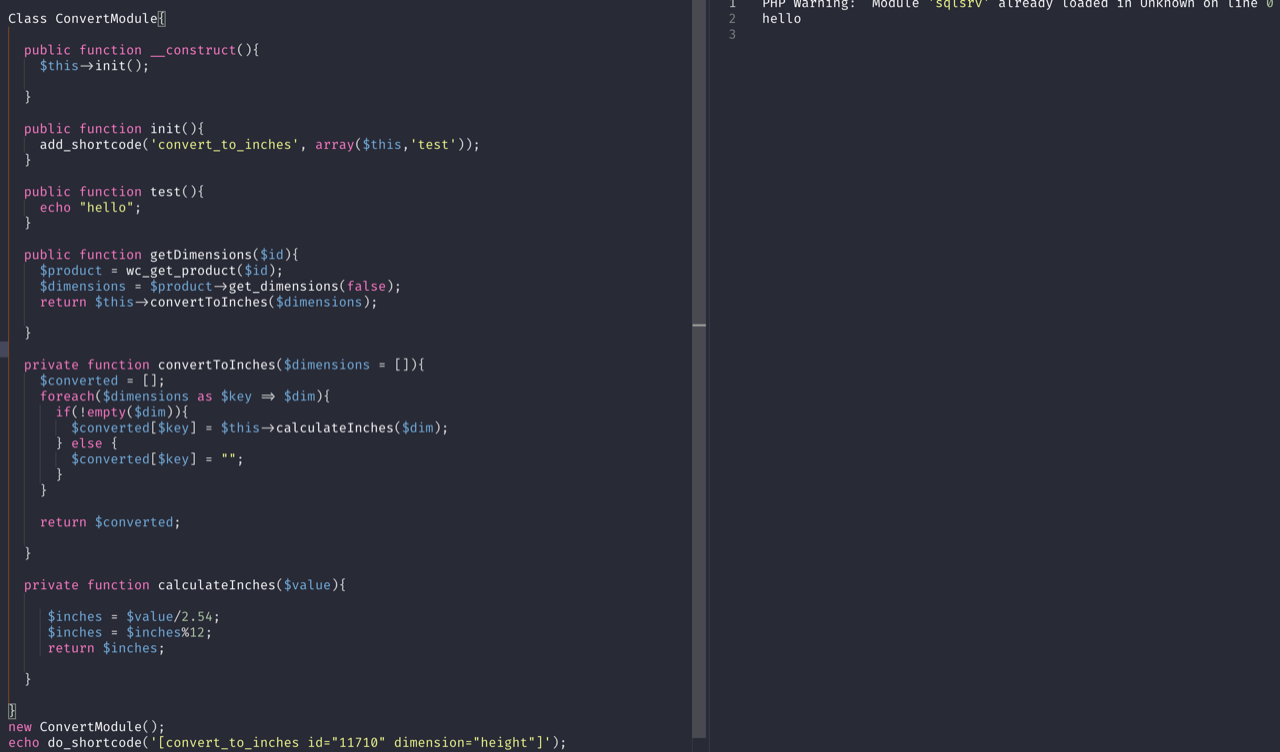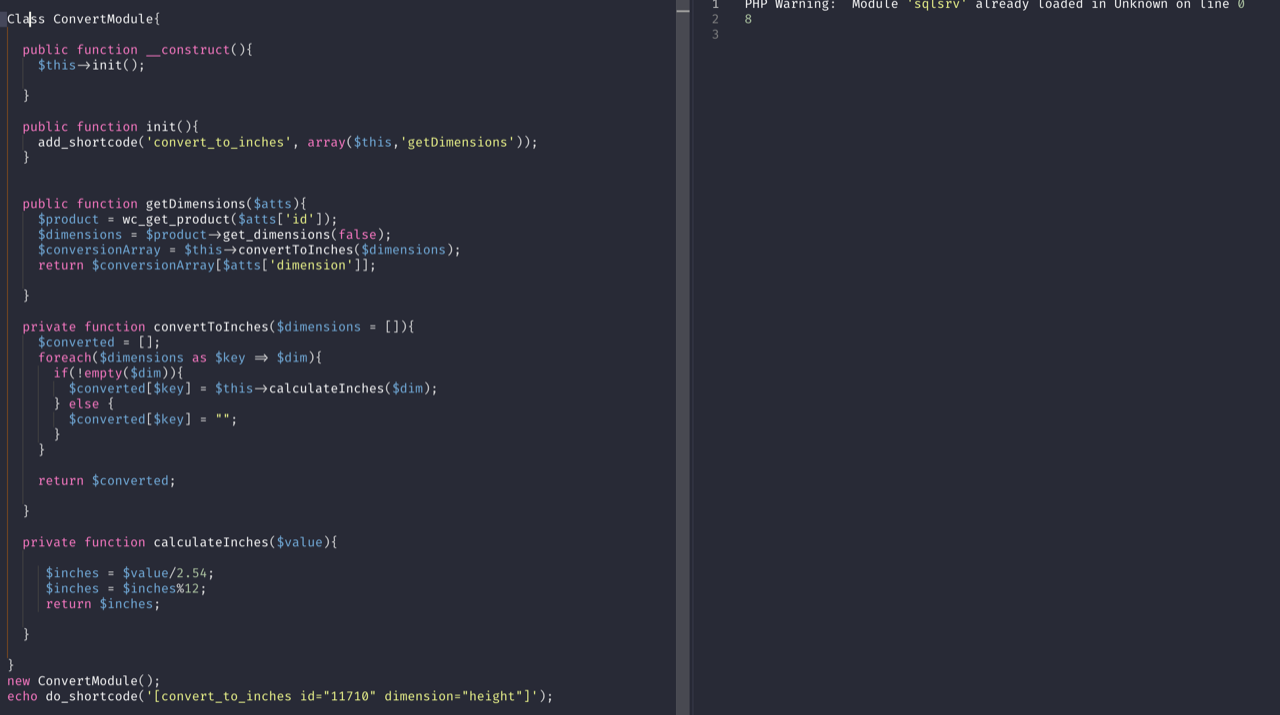Tinkerwell 3 is here!Tinkerwell
Go back to Blog

# Wordpress plugin development with Tinkerwell

This is a case study by Martin from smbcoach.ca.

In this article about plugin development for Wordpress with Tinkerwell, we're using Tinkerwell to create a prototype of the plugin before we change the project itself. This helps us sketching out the plugin within minutes and understand the effort that this plugin requires. It also allows us to use the live site as our playground because we don't change any code on the site but can still use the data of the site without setting up a staging environment or copy data to an existing one.

The to be written plugin creates a shortcode that converts the size in centimeters of a WooCommerce product to inches. That's actually a pretty simply function but as long as we don't know exactly where the original information for the product is stored, we have to debug our way to this information – and doing this on the live site would throw errors for our visitors.

So at first, we're starting off with a class skeleton and getting a product loaded.

``````class ConvertModule
{
public function test(\$id)
{
\$product = wc_get_product(\$id);

return \$product;
}
}

\$convert = new ConvertModule();
print_r(\$convert->test(11710));
``````This product class gets us a peak into the object, but also shows us that it's too much data that we don't care about. So when needing the product dimensions, we can look at the WooCommerce docs and see that we have access to a method that gets the dimensions for a product. As you never know what an object in Wordpress returns, let's have a peak.

``````class ConvertModule
{
public function getDimensions(\$id)
{
\$product = wc_get_product(\$id);
\$dimensions = \$product->get_dimensions();

return \$dimensions;
}
}

\$convert = new ConvertModule();
print_r(\$convert->getDimensions(11710));
``````

OK it looks like this `get_dimensions()` method seems to return a deprecation error:

``````PHP Deprecated: WC_Product::get_dimensions was called with an argument that is <strong>deprecated</strong> since version 3.0! By default, get_dimensions has an argument set to true so that HTML is returned. This is to support the legacy version of the method. To get HTML dimensions, instead use wc_format_dimensions() function. Pass false to this method to return an array of dimensions.
``````

Fair enough. The function `wc_format_dimensions()` seems to return the HTML version of the dimensions and this is not what we want. We'd like to get the data version so we can then get some math done. Looking at the functions params, it looks like we can pass an argument to the method to get an array version.

``````class ConvertModule
{
public function getDimensions(\$id)
{
\$product = wc_get_product(\$id);
\$dimensions = \$product->get_dimensions(false);

return \$dimensions;
}
}

\$convert = new ConvertModule();
print_r(\$convert->getDimensions(11710));
``````All right! We're making progress and get the data that we need:

``````Array
(
[length] =>
[width] => 15.25
[height] => 21.50
)
``````

Let's augment our class to include the actual conversion and see what we get. Within a quick search on the internet, we get the math we need to do to get from CM to Inches.

``````class ConvertModule
{
public function getDimensions(\$id)
{
\$product = wc_get_product(\$id);
\$dimensions = \$product->get_dimensions(false);

return \$this->convertToInches(\$dimensions);
}

private function convertToInches(\$dimensions = [])
{
\$converted = [];
foreach (\$dimensions as \$key => \$dim) {
if (empty(\$dim)) continue;

\$converted[\$key] = \$this->calculateInches(\$dim);
}

return \$converted;
}

private function calculateInches(\$value)
{
\$inches = \$value / 2.54;
\$inches = \$inches % 12;
return \$inches;
}
}

\$convert = new ConvertModule();
print_r(\$convert->getDimensions(11710));

``````

Gives us an array with converted data:

``````Array
(
[length] => 0
[width] => 6
[height] => 8
)
``````

In the code above we added two methods to call a conversion function then do the actual math. Eventually we could make it a more universal class where we could convert any units to other units but this is a prototype for now.

Next, let's add a shortcode to call it from within a product details template or a post. The shortcode should look like this:

``````[convert_to_inches id="11710" dimension="height"]
``````

Let's start by testing that we can get the shortcode fired with a simple echo function and none of the parameters:

``````class ConvertModule
{
public function __construct()
{
\$this->init();
}

public function init()
{
}

public function test()
{
echo "hello";
}

public function getDimensions(\$id)
{
\$product = wc_get_product(\$id);
\$dimensions = \$product->get_dimensions(false);

return \$this->convertToInches(\$dimensions);
}

private function convertToInches(\$dimensions = [])
{
\$converted = [];
foreach (\$dimensions as \$key => \$dim) {
if (empty(\$dim)) continue;

\$converted[\$key] = \$this->calculateInches(\$dim);
}

return \$converted;
}

private function calculateInches(\$value)
{
\$inches = \$value / 2.54;
\$inches = \$inches % 12;
return \$inches;
}
}

new ConvertModule();
echo do_shortcode('[convert_to_inches id="11710" dimension="height"]');

``````

This gives us `Hello` 🙂Ok let's see if we can to use the parameters now. The method that the shortcode is associated to receives an attributes Array (\$atts). So in our case we would get access to \$atts['id'] and \$atts['dimension'] to use in our methods.``````class ConvertModule
{
public function __construct()
{
\$this->init();
}

public function init()
{
}

public function getDimensions(\$atts)
{
\$product = wc_get_product(\$atts['id']);
\$dimensions = \$product->get_dimensions(false);
\$conversionArray = \$this->convertToInches(\$dimensions);
return \$conversionArray[\$atts['dimension']];
}

private function convertToInches(\$dimensions = [])
{
\$converted = [];
foreach (\$dimensions as \$key => \$dim) {
if (empty(\$dim)) continue;

\$converted[\$key] = \$this->calculateInches(\$dim);
}

return \$converted;
}

private function calculateInches(\$value)
{
\$inches = \$value / 2.54;
\$inches = \$inches % 12;
return \$inches;
}
}

new ConvertModule(); // this simply loads the class in Tinkerwell. In the final plugin, we would handle this in the autoloader of the plugin.

echo do_shortcode('[convert_to_inches id="11710" dimension="height"]');

``````

The shortcode calls for height dimensions for product id 11710 and outputs 8, it looks like we have a lift off 🙂

Now we have a good idea on how we can get this plugin built, and what kind of data and parameters are needed. We can now scope the project pretty accurately, identify where we will need checks and defaults values and already have a prototype running.

## Tinkerwell: The code runner for PHP

The must-have companion to your favorite IDE. Quickly iterate on PHP code within the context of your web application.